# Finding Reduced Basis for LatticesPage 2

#### WATCH ALL SLIDES

For given positive

Do there exist such that:

(is s a subset sum of the mi’s)?

Slide 15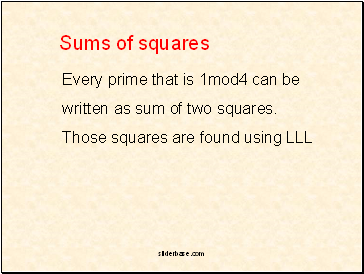## Sums of squares

Every prime that is 1mod4 can be

written as sum of two squares.

Those squares are found using LLL

Slide 16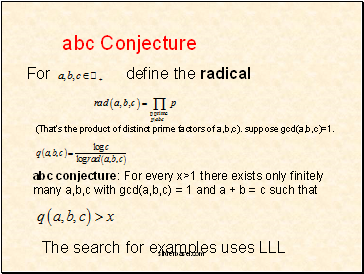## abc Conjecture

(That’s the product of distinct prime factors of a,b,c). suppose gcd(a,b,c)=1.

abc conjecture: For every x>1 there exists only finitely many a,b,c with gcd(a,b,c) = 1 and a + b = c such that

The search for examples uses LLL

Slide 17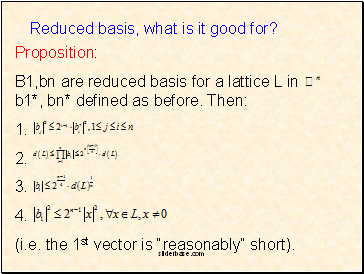Proposition:

B1,bn are reduced basis for a lattice L in b1*, bn* defined as before. Then:

1.

2.

3.

4.

(i.e. the 1st vector is “reasonably” short).

Reduced basis, what is it good for?

Slide 18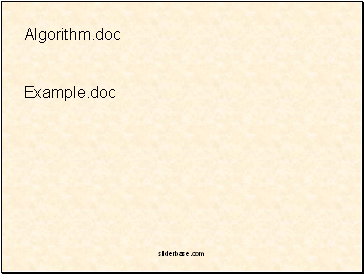Algorith.doc

Example.doc

Slide 19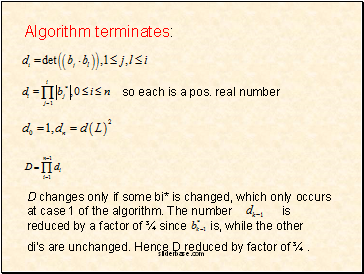Algorith terminates:

so each is a pos. real number

D changes only if some bi* is changed, which only occurs at case 1 of the algorith. The number is reduced by a factor of ¾ since is, while the other

di’s are unchanged. Hence D reduced by factor of ¾ .

Slide 20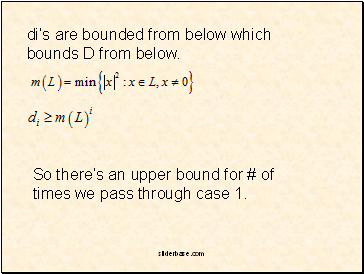di’s are bounded from below which bounds D from below.

So there’s an upper bound for # of times we pass through case 1.

Slide 21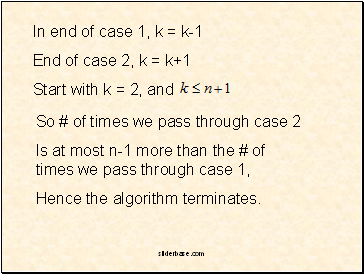In end of case 1, k = k-1

End of case 2, k = k+1

So # of times we pass through case 2

Is at most n-1 more than the # of times we pass through case 1,

Hence the algorith terminates.

Slide 22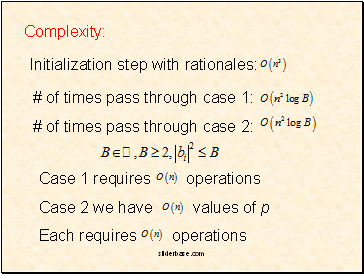Complexity:

Initialization step with rationales:

# of times pass through case 1:

# of times pass through case 2:

Case 1 requires operations

Case 2 we have values of p

Each requires operations

Slide 23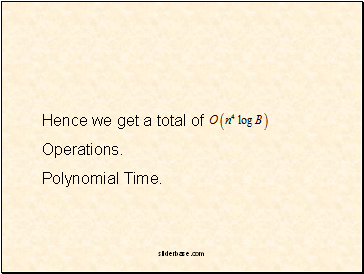Hence we get a total of

Operations.

Polynomial Time.

Go to page:
1  2